## Abstracts

1. An inequality of the Lupas type for Cebysev functionals is established.

2. Some sums of series of Rogers dilogarithm functions are established by Abel's functional equation.

3. In this article, for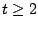, a general generalization of Jordan's inequality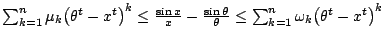for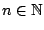and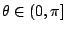is established, where the coefficients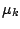and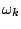defined by recursing formulas (11) and (12) are the best possible. As an application, L. Yang's inequality is refined.

4. In this short paper, it is proved that the function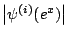for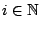is subadditive in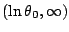and superadditive in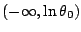, where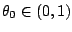is the unique root of equation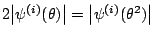.

5. In this article, van der Corput's inequality is generalized by using the well known Euler-Maclaurin sum formula and other analytic techniques.

6. Sharp error estimates in approximating the Stieltjes integral with bounded integrands and bounded integrators respectively, are given. Applications for three point quadrature rules of n-time differentiable functions are also provided.

7. An approximation of the Stieltjes integral of bounded integrals and continuous integrators via the Darst-Pollard inequality is given. Applications for the generalised trapezoid formula and the Ostrowski inequality for functions of bounded variation are also provided.

8. Some inequalities related to the Hölder integral inequality and applications for bounding the Cebysev functional are given.

9. An iterative approach is used to represent multidimensional integrals in terms of lower dimensional integrals and function evaluations. The procedure is quite general utilising one dimensional identities as the generator to procure multidimensional identities. Bounds are obtained from the identities. Both weighted and unweighted integrals are considered.

10. Upper and lower bounds for the norm of a linear combination of vectors are given. Applications in obtaining various inequalities for the quantities  || x/||x|| -y||y|| || and || x/||y|| -y||x|| || , where x and y are nonzero vectors, that are related to the Massera-Schäffer and the Dunkl-Williams inequalities are also provided. Some bounds for the unweighted Cebysev functional are given as well.

11. New upper and lower bounds for the p-angular distance in normed linear spaces are given. Some of the obtained upper bounds are better than the corresponding results due to L. Maligranda recently established in the paper [Simple norm inequalities, Amer. Math. Monthly, 113 (2006), 256-260].

12. In this paper, we introduce 3-dimensional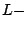summing method, which is a rearrangement of the summation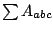with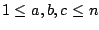. Applying this method on some special arrays, we obtain some identities on the Riemann zeta function and digamma function. Also, we give a Maple program for this method to obtain identities with input various arrays and out put identities concerning some elementary functions and hypergeometric functions. Finally, we introduce a further generalization ofsumming method in higher dimension spaces.

1. Some inequalities in normed algebras that provides lower and upper bounds for the norm of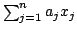are obtained. Applications for estimating the quantities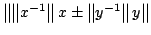and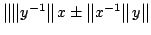for invertible elements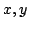in unital normed algebras are also given.

2. An operator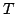is called-normal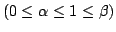if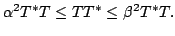In this paper, we establish various inequalities between the operator norm and its numerical radius of-normal operators in Hilbert spaces. For this purpose, we employ some classical inequalities for vectors in inner product spaces.

3. A refinement of the discrete Jensen's inequality for convex functions defined on a convex subset in linear spaces is given. Application for f-divergence measures including the Kullback-Leibler and Jeffreys divergences are provided as well.

4. A new sharp bound of the Cebysev functional for the Riemann-Stieltjes integral is obtained. Applications for quadrature rules including the trapezoid and mid-point rule are given.

5. Sharp bounds for the deviation of a real-valued function f defined on a compact interval [a,b] to the chord generated by its end points (a, f(a)) and (b, f(b))  under various assumptions for f and f' including absolute continuity, convexity, bounded variation, monotonicity etc., are given. Some applications for weighted means and f-divergence measures in Information Theory are also provided.

6. Some new sharp upper bounds for the absolute value of the error functional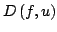in approximating the Riemann-Stieltjes integral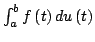by the quantity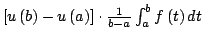are given.